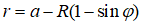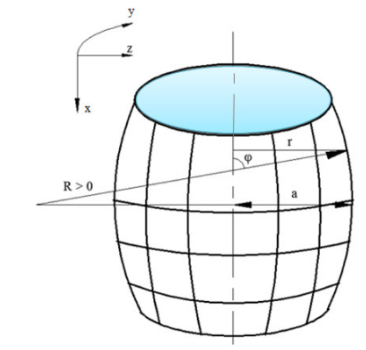## How to simplify expression with RootOf...

I have a complicated expression which involves RootOf. How to get rid of and simpliy the equation?

I am looking for an expression for eta(H) not a numerical value.

## How to code a conjecture?...

Hello,

I am working on a project to code a conjecture in Maple and I am stuck.  It is as follows:

"Someone had a conjecture that any odd number greater than one can be written as the sum of a prime number and twice a perfect square.  (ie 3=3+2*02, 15 = 7+2*22)  I would like to know if this holds for all the numbers from 3 to 9,000 and if not, which odd numbers fail this criteria."

I have very little coding experience so I am looking to see if anyone has an idea where I can go with this.  I tried the following:

X=P+2*N where

X:=seq(3+2i, i=0..4499);

P:={seq(ithprime(i), i=1..1250)};

N:=seq(i^2, i=0..100);

Once I get this far, however, I don't know where to go.  I would like the left side of the equation to run from 0 through 4499 (giving me a value of over 9000) while the right side does the same thing.  If there are any situations where the left side and right side do not equal each other, I want it to tell me.

My apologies if this is too wordy or confusing.  Thank you.

## How to find number a, b, c, d, m so that this equa...

I  am trying to find the integer numbers `a, b, c, d, m` so that the equation

(x−a)(x−b)(x−c)(x−d)=m

where a+d=b+c  and m≠0 has four integer solutions.

I found this equation randomly
solve((x-5) (x-3) (x+6) (x+8)=504, x);
How to find number a, b, c, d, m so that the equation
(x−a)(x−b)(x−c)(x−d)=m
has four integer solutions?

## Rules to force [X,X] = 0 ?...

I am still a novice of Maple, but I want to carry out recurvive applications of some formula that involves non-commutative operators X and Y ([X,Y] = XY-YX). I used the following code, but I do not know how to tell Maple a rule that [X,X] = [Y,Y] = 0:

```with(Physics);
Setup(mathematicalnotation = true);
Setup(noncommutativeprefix = {X, Y});

Z := X + Y + (1/2)*Commutator(X,Y);
subs(X=Y,Z);```

The output is like this:

```> Z := X + Y + (1/2)*Commutator(X,Y);
Z := X + Y + 1/2 [X, Y][-]

> subs(X=Y,Z);
2 Y + 1/2 [Y, Y][-]```

The above [Y,Y] must be interpreted as zero, of course.

Thank you very much.

Hello,

How I can Plot this structure using define new coordinate such as cylinderical om\ne or addcoords?plot.mw

## By doing manual calculations the solutions doesn't...

test1_03.mw

The solution I'm getting here for v(y), doesn't satisfy my original two equations. Like for example if I choose u(y)=y, for easy check it can be seen that my de2 equation doesn't satisfy that value.

Can anyone help me out?

## How to find the integer numbers a, b, c, d, e, f, ...

I see this question at here
https://mathematica.stackexchange.com/questions/239874/how-to-reduce-timing-to-find-the-integer-numbers-a-b-c-d-e-f-g-h-k-m-of

How to solve this problem by Maple?

## How to take off a term in XY...

I want to remove the term XY
f:=(x,y)->2*x²+xy+y²+4x-y-2=0;
eq := simplify(subs(x = X-9/7, y = Y+8/7, f(x, y)));
theta:=Pi/8;
ex := simplify(subs(X = cos(theta)*X-sin(theta)*Y, Y = sin(theta)*X+cos(theta)*Y, eq)); evalf(%);
How to obtain (3+sqrt(2))/2*X²+(3-sqrt(2))/2=36 ? Thank you.

## How to reduce timing to solve this equation?...

I am trying to solve this equation, I tried

```restart;
ListTools[Categorize];
L := [];
for a to 20 do
for b to 20 do
for c to 20 do
for d to 20 do
for e to 20 do
for f to 20 do
for g to 20 do
for h to 20 do
if sqrt(a+b*sqrt(c+d*sqrt(e +f*sqrt(g)))) = h then L := [op(L), [a, b, c, d, e, f, g, h]] end if
end do end do end do end do end do end do end do end do; nops(L)
```

where g is not a square of a integer number . I do not get any solutions for a long time. How to reduce timing to solve this equation?

## Why can't Maple solve a simple equation...

This question came to me when I answered in this thread  https://www.mapleprimes.com/questions/231603-Solve-Onevariable-Equation

The equation there is quite cumbersome, I extracted a shorter subexpression from it (I converted all floats to exact constants), but the  solve  command hangs when trying to solve it. fsolve  handles the equation easily. We can see that the equation  Eq  is quite simple and easy to solve even by hand. I ask this question in a separate topic, because this seems to be a serious bug in the  solve  command.

```restart;
Eq:=1-1/(1+203808*exp(-342569/506*t)*(1/131537))^(131537/203808)=44983/56599;
solve(Eq);```Edit. I noticed that the  isolate  command solves the problem, but of course the question remains open with  solve .

## Absolute ploting in dsolve...

How to plot absolute value in dsolve?
absolute_plot.mw

Plotting in the first coordinate in the plane......

## How to create a tetrahedron whose vertices are int...

I know that, tetrahedron with vertices (0, 0, 0), (3, 0, 0), (0, 5, 0), (0, 0, 14) has center of insphere is  (1, 1, 1) and radius R = 1. How to create a tetrahedron whose coordinates of all vertices are integers, the inscribed spherical center and the inscribed spherical radius are integers?

## what does "Warning, data could not be converted to...

restart; _local(D); A := [-L, 0]; B := [L, 0]; C := [x, y]; D := [-x, y]; Dist := proc (X, Y) options operator, arrow; sqrt((X-Y)^2+(X-Y)^2) end proc; Eq := (Dist(C, D) = Dist(C, B))^2; centre := [solve(diff(Eq, x), x), solve(diff(Eq, y), y)]; with(geometry); `assuming`([conic(p, Eq, [x, y])], [L > 0]); detail(p); asymptotes(p); y_acymp := `~`[solve](`~`[Equation](asymptotes(p)), y); y := solve(Eq, y) P := proc (X, L0) local Curve, Asymptote, Trapezoid, T, pt, Ip; Curve := plot(eval([y, -y], L = L0), x = -(1/3)*L0 .. 15, color = red, thickness = 3); Asymptote := plot(eval([-sqrt(3)*x-(1/3)*sqrt(3)*L, sqrt(3)*x+(1/3)*sqrt(3)*L], L = L0), x = -(1/3)*L0 .. 15, linestyle = 3, color = black, thickness = 0); Trapezoid := plottools:-polygon(eval([A, B, C, D], [L = L0, x = X]), color = "LightGreen"); Ip := (1/3)*(eval(C, [x = X-(1/3)*L0, L = L0])); #centre of gravity of ABC T := plots:-textplot([[(eval(A, [x = X, L = L0]))[], "A"], [(eval(B, [x = X, L = L0]))[], "B"], [(eval(C, [x = X, L = L0]))[], "C"], [(eval(D, [x = X, L = L0]))[], "D"], [Ip[], "I"]], align = {above, left}, font = [TIMES, 16]); pt := plot([A, B, C, Ip], style = plottools:-point, color = blue, symbolsize = 15); plots:-display(Curve, Asymptote, Trapezoid, T, pt, scaling = constrained, size = [400, 800]) end proc; a := 7; P((1/2)*a, 6) I would like of location of centre of gravity of ABC. Thank you.

## How can I reduce the time to solve this is system ...

This  is a question from https://mathematica.stackexchange.com/questions/239055/how-to-reduce-the-time-to-solve-this-system-of-equations. How to reduce the time in Maple?

``````  {x, y, z, a, b, m, n} /. Solve[{1/x + 1/y == 1/z, a/x  + b/y == m/n, 2 <= x <= 30, 2 <= y <= 30, 1 <= z <= 30, 2 <= a <= 10, 2 <= b <= 10, a > b, 2 <= m <= 10, 2 <= n <= 10, x > y, GCD[m, n] == 1, GCD[a, b, m] == 1, m <= n}, {x, y, z, a, b, m, n}, Integers]
``````